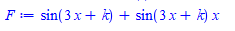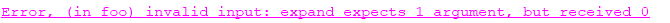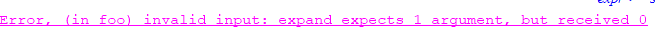# Question:how to make forget(expand) work in a procedure?

## Question:how to make forget(expand) work in a procedure?

Maple 2021

I was expanding an expressions, and found that Maple expands trig also. Then found there is a way to turn that off. Which is great. I tested it in my worksheet and it works. But when I put the same code inside my module, I get an error.  It seems like some scoping issue which I do not understand.

Here is a MWE

```restart;
expr:=(1+x)*sin(3*x+k);
forget(expand);
expand(expandoff());
expandoff(cos,sin,exp);
F:=expand(expr);
forget(expand);
```

which givesWhich does what I want. But inside a proc, the code fails

```foo:=proc(expr)
local F;

forget(expand);
expand(expandoff());
expandoff(cos,sin,exp);
F:=expand(expr);
forget(expand);

end proc;
```

And now when calling it as

```expr:=x*sin(3*x+k);
foo(expr)
```What Am I doing wrong? And how to make it work? I need to prevent expand() from expanding these functions. Help says that expand has memory table, and I want to clear that before and after doing this, so it does not affect later code.

Maple 2021.1

Update

I just found out, if I add a restart before defining my function, then the error goes away

```restart;
expr:=(1+x)*sin(3*x+k);
forget(expand);
expand(expandoff());
expandoff(cos,sin,exp);
F:=expand(expr);
forget(expand);

foo:=proc(expr)
local F;

forget(expand);
expand(expandoff());
expandoff(cos,sin,exp);
F:=expand(expr);
forget(expand);
end proc;

#
expr:=(1+x)*sin(3*x+k);
foo(expr)

#no error. now it works

```

I need this to work repeatedly inside a module, so I can't do restart each time ofcourse like the above.

Even if I do restart before defining the function, next time I call it, it will fail:

```restart;
foo:=proc(expr)
local F;

forget(expand);
expand(expandoff());
expandoff(cos,sin,exp);
F:=expand(expr);
forget(expand);
end proc;

#
expr:=(1+x)*sin(3*x+k);
foo(expr); #OK
foo(expr); #FAILED
```Very strange.﻿